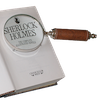#### You may also like### Number Round Up

Arrange the numbers 1 to 6 in each set of circles below. The sum of each side of the triangle should equal the number in its centre.### Number Detective

Follow the clues to find the mystery number.### Grouping Goodies

Pat counts her sweets in different groups and both times she has some left over. How many sweets could she have had?

# Venn Diagrams

##### Age 5 to 11Challenge Level

Where would you put a number which is a multiple of 5 but not even?
Where would an even multiple of 5 go?
Where would a number which is odd but not a multiple of 5 go?
Where would you put a number which is not a multiple of 5 and not even?